# Formulary (plasmapy.formulary)

plasmapy.formulary provides theoretical formulas for calculation of physical quantities helpful for plasma physics.

The subpackage makes heavy use of Quantity for handling conversions between different unit systems. This is especially important for electron-volts, commonly used in plasma physics to denote temperature, although it is technically a unit of energy.

Most functions expect Quantity objects as inputs, however some will use the validate_quantities decorator to automatically cast arguments to Quantity objects with the appropriate units. If that happens, you will be notified via a astropy.units.UnitsWarning.

Please note that well-maintained physical constant data with units and uncertainties can be found in astropy.constants.

## Examples

For a general overview of how unit-based input works, take a look at the following examples: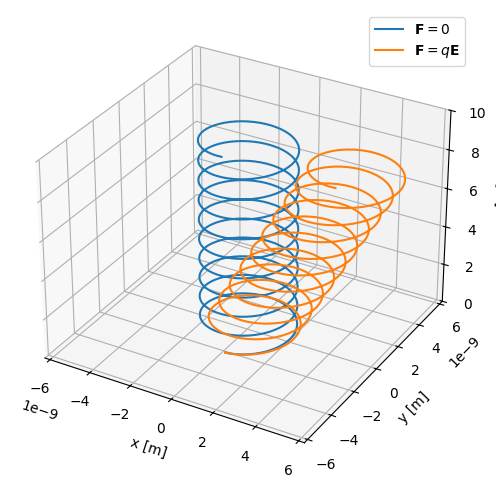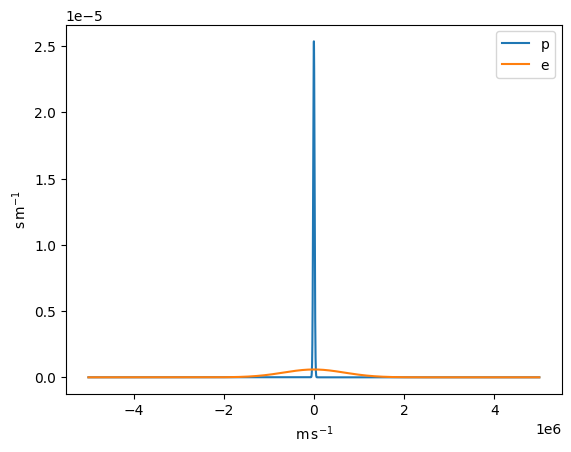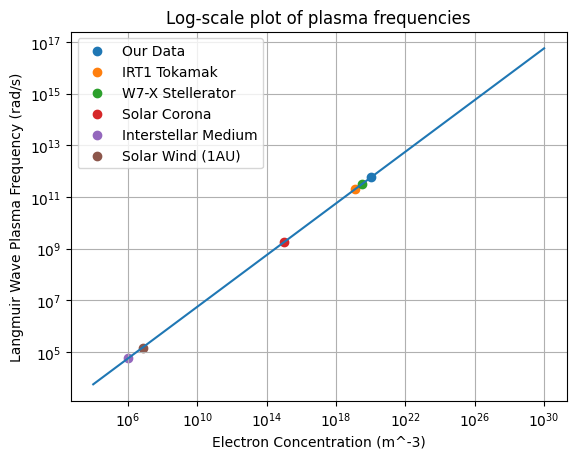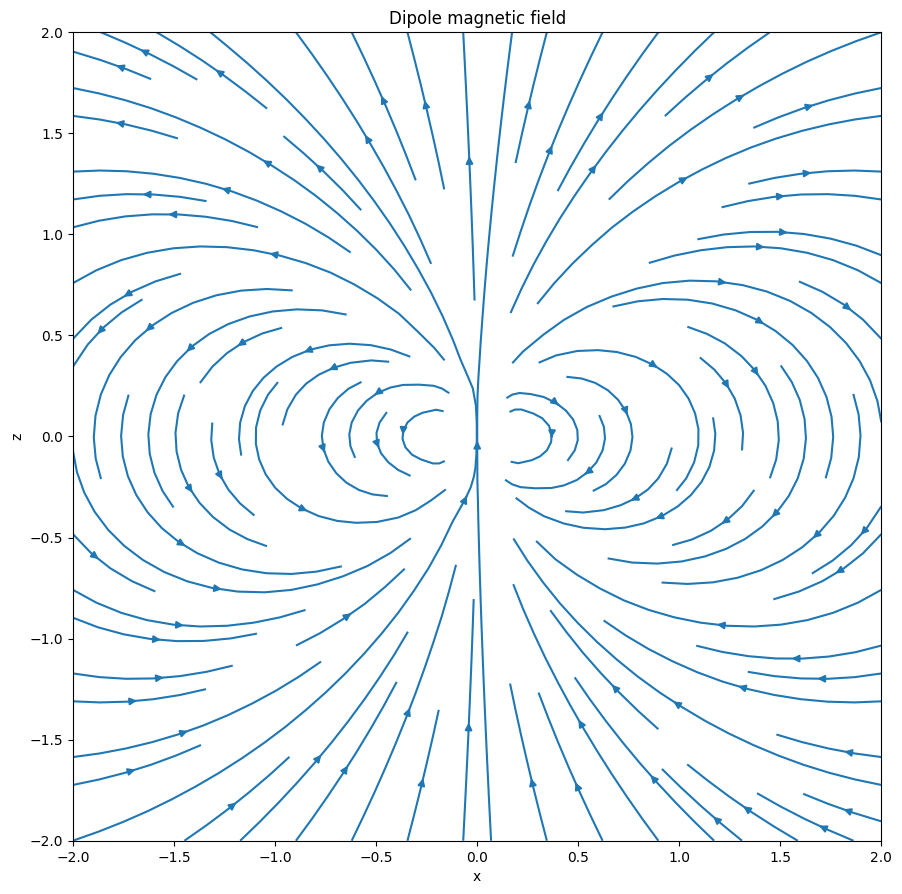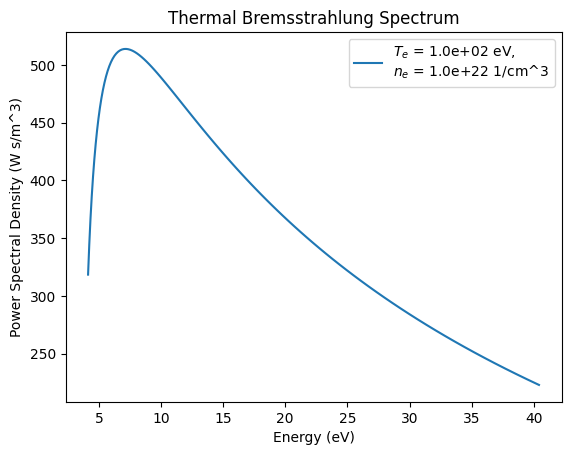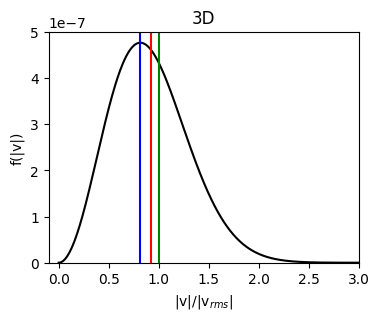## API

The formulary subpackage contains commonly used formulae from plasma science.

### Sub-Packages & Modules

 braginskii Functions to calculate classical transport coefficients. collisions Functions to calculate transport coefficients. densities Functions to calculate plasma density parameters. dielectric Functions to calculate plasma dielectric parameters dimensionless Module of dimensionless plasma parameters. distribution Common distribution functions for plasmas, such as the Maxwellian or Kappa distributions. drifts Functions for calculating particle drifts. frequencies Functions to calculate fundamental plasma frequency parameters. ionization Functions related to ionization states and the properties thereof. lengths Functions to calculate fundamental plasma length parameters. magnetostatics Define MagneticStatics class to calculate common static magnetic fields as first raised in issue #100. mathematics Mathematical formulas relevant to plasma physics. misc Functions for miscellaneous plasma parameter calculations. parameters quantum Functions for quantum parameters, including electron degenerate gases and warm dense matter. radiation Functions for calculating quantities associated with electromagnetic radiation. relativity Functions for calculating relativistic quantities ($$v \to c$$). speeds Functions to calculate fundamental plasma speed parameters.

### Classes

 CircularWire(normal, center, radius, current) Circular wire(coil) class. ClassicalTransport(T_e, n_e, T_i, n_i, ion, m_i) Classical transport coefficients (e.g. FiniteStraightWire(p1, p2, current) Finite length straight wire class. GeneralWire(parametric_eq, t1, t2, current) General wire class described by its parametric vector equation InfiniteStraightWire(direction, p0, current) Infinite straight wire class. MagneticDipole(moment, p0) Simple magnetic dipole — two nearby opposite point charges. Abstract class for all kinds of magnetic static fields Compute collision frequencies between two slowly flowing Maxwellian populations. RelativisticBody(particle, ...) A physical body that is moving at a velocity relative to the speed of light. Compute collision frequencies between test particles (labeled 'a') and field particles (labeled 'b'). Abstract wire class for concrete wires to be inherited from.### Functions

 Alfven_speed(B, density[, ion, z_mean]) Calculate the Alfvén speed. beta(T, n, B) Compute the ratio of thermal pressure to magnetic pressure. Bohm_diffusion(T_e, B) Return the Bohm diffusion coefficient. chemical_potential(n_e, T) Calculate the ideal chemical potential. cold_plasma_permittivity_LRP(B, species, n, ...) Magnetized cold plasma dielectric permittivity tensor elements. cold_plasma_permittivity_SDP(B, species, n, ...) Magnetized cold plasma dielectric permittivity tensor elements. collision_frequency(T, n, species[, z_mean, ...]) Coulomb_cross_section(impact_param) Cross section for a large angle Coulomb collision. Coulomb_logarithm(T, n_e, species[, z_mean, ...]) Compute the Coulomb logarithm. coupling_parameter(T, n_e, species[, ...]) Ratio of the Coulomb energy to the kinetic (usually thermal) energy. critical_density(omega) Calculate the plasma critical density for a radiation of a given frequency. deBroglie_wavelength(V, particle) Return the de Broglie wavelength. Debye_length(T_e, n_e) Calculate the characteristic decay length for electric fields, Debye_number(T_e, n_e) Return the number of electrons within a sphere with a radius of the Debye length. diamagnetic_drift(dp, B, n, q) Calculate the diamagnetic fluid perpendicular drift. electron_thermal_conductivity(T_e, n_e, T_i, ...) Calculate the thermal conductivity for electrons. electron_viscosity(T_e, n_e, T_i, n_i, ion) Calculate the electron viscosity. ExB_drift(E, B) Calculate the "electric cross magnetic" particle drift. Calculate the kinetic energy in a degenerate electron gas. Fermi_integral(x, j) Calculate the complete Fermi-Dirac integral. force_drift(F, B, q) Calculate the general force drift for a particle in a magnetic field. Average momentum relaxation rate for a slowly flowing Maxwellian distribution of electrons. fundamental_ion_collision_freq(T_i, n_i, ion) Average momentum relaxation rate for a slowly flowing Maxwellian distribution of ions. gyrofrequency(B, particle[, signed, Z, to_hz]) Calculate the particle gyrofrequency in units of radians per second. gyroradius(B, particle, *[, Vperp, T_i, T]) Return the particle gyroradius. Hall_parameter(n, T, B, ion, particle[, ...]) Calculate the particle Hall parameter for a plasma. impact_parameter(T, n_e, species[, z_mean, ...]) Impact parameters for classical and quantum Coulomb collision. impact_parameter_perp(T, species[, V]) Distance of closest approach for a 90° Coulomb collision. inertial_length(n, particle) Calculate a charged particle's inertial length. ion_sound_speed(T_e, T_i, ion[, n_e, k, ...]) Return the ion sound speed for an electron-ion plasma. ion_thermal_conductivity(T_e, n_e, T_i, n_i, ion) Calculate the thermal conductivity for ions. ion_viscosity(T_e, n_e, T_i, n_i, ion[, ...]) Calculate the ion viscosity. ionization_balance(n, T_e) Return the average ionization state of ions in a plasma assuming that the numbers of ions in each state are equal. kappa_thermal_speed(T, kappa, particle[, method]) Return the most probable speed for a particle within a Kappa distribution. kappa_velocity_1D(v, T, kappa[, particle, ...]) Return the probability density at the velocity v in m/s to find a particle particle in a plasma of temperature T following the Kappa distribution function in 1D. kappa_velocity_3D(vx, vy, vz, T, kappa[, ...]) Return the probability density function for finding a particle with velocity components v_x, v_y, and v_zin m/s in a suprathermal plasma of temperature T and parameter kappa which follows the 3D Kappa distribution function. Knudsen_number(characteristic_length, T, ...) Knudsen number (dimensionless). Return the Lorentz factor. lower_hybrid_frequency(B, n_i, ion[, to_hz]) Return the lower hybrid frequency. Lundquist_number(L, B, density, sigma[, ...]) Compute the Lundquist number Mag_Reynolds(U, L, sigma) Compute the magnetic Reynolds number Calculate the magnetic energy density. Calculate the magnetic pressure. mass_density(density, particle[, z_ratio]) Calculate the mass density from a number density. Maxwellian_1D(v, T[, particle, v_drift, ...]) Probability distribution function of velocity for a Maxwellian distribution in 1D. Maxwellian_speed_1D(v, T[, particle, ...]) Probability distribution function of speed for a Maxwellian distribution in 1D. Maxwellian_speed_2D(v, T[, particle, ...]) Probability distribution function of speed for a Maxwellian distribution in 2D. Maxwellian_speed_3D(v, T[, particle, ...]) Probability distribution function of speed for a Maxwellian distribution in 3D. Maxwellian_velocity_2D(vx, vy, T[, ...]) Probability distribution function of velocity for a Maxwellian distribution in 2D. Maxwellian_velocity_3D(vx, vy, vz, T[, ...]) Probability distribution function of velocity for a Maxwellian distribution in 3D. mean_free_path(T, n_e, species[, z_mean, V, ...]) Collisional mean free path (m). mobility(T, n_e, species[, z_mean, V, method]) Return the electrical mobility. permittivity_1D_Maxwellian(omega, kWave, T, ...) Compute the classical dielectric permittivity for a 1D Maxwellian plasma. plasma_frequency(n, particle[, z_mean, to_hz]) Calculate the particle plasma frequency. quantum_theta(T, n_e) Compare Fermi energy to thermal kinetic energy to check if quantum effects are important. Calculate the relativistic energy (in joules) of an object of mass m and velocity v. resistivity(T_e, n_e, T_i, n_i, ion[, m_i, ...]) Calculate the resistivity. Reynolds_number(rho, U, L, mu) Compute the Reynolds number. rot_a_to_b(a, b) Calculates the 3D rotation matrix that will rotate vector a to be aligned with vector b. Saha(g_j, g_k, n_e, E_jk, T_e) Return the ratio of populations of two ionization states. Spitzer_resistivity(T, n, species[, z_mean, ...]) Spitzer resistivity of a plasma. thermal_bremsstrahlung(frequencies, n_e, T_e) Calculate the bremsstrahlung emission spectrum for a Maxwellian plasma in the Rayleigh-Jeans limit $$ℏ ω ≪ k_B T_e$$ Calculate the thermal de Broglie wavelength for electrons. Return the thermal pressure for a Maxwellian distribution. thermal_speed(T, particle[, method, mass, ndim]) Calculate the speed of thermal motion for particles with a Maxwellian distribution. thermal_speed_coefficients(method, ndim) Get the thermal speed coefficient corresponding to the desired thermal speed definition. thermoelectric_conductivity(T_e, n_e, T_i, ...) Calculate the thermoelectric conductivity. Calculate the exponential scale length for charge screening for cold and dense plasmas. upper_hybrid_frequency(B, n_e[, to_hz]) Return the upper hybrid frequency. Calculate the Wigner-Seitz radius, which approximates the inter-particle spacing.

### Aliases

PlasmaPy provides aliases of the most common plasma functionality for user convenience. Aliases in PlasmaPy are denoted with a trailing underscore (e.g., alias_). For further details, please refer to the contributor guide’s section on aliases.

 betaH_(n, T, B, ion, particle[, ...]) Alias to Hall_parameter. cs_(T_e, T_i, ion[, n_e, k, gamma_e, ...]) Alias to ion_sound_speed. cwp_(n, particle) Alias to inertial_length. DB_(T_e, B) Alias to Bohm_diffusion. Ef_(n_e) Alias to Fermi_energy. lambdaD_(T_e, n_e) Alias to Debye_length. lambdaDB_(V, particle) Alias to deBroglie_wavelength. nD_(T_e, n_e) Alias to Debye_number. oc_(B, particle[, signed, Z, to_hz]) Alias to gyrofrequency. Alias to magnetic_pressure. pth_(T, n) Alias to thermal_pressure. rc_(B, particle, *[, Vperp, T_i, T]) Alias to gyroradius. Re_(rho, U, L, mu) Alias to Reynolds_number. rho_(density, particle[, z_ratio]) Alias to mass_density. rhoc_(B, particle, *[, Vperp, T_i, T]) Alias to gyroradius. Rm_(U, L, sigma) Alias to Mag_Reynolds. Alias to magnetic_energy_density. va_(B, density[, ion, z_mean]) Alias to Alfven_speed. vd_(dp, B, n, q) Alias to diamagnetic_drift. veb_(E, B) Alias to ExB_drift. vfd_(F, B, q) Alias to force_drift. vth_(T, particle[, method, mass, ndim]) Alias to thermal_speed(). vth_kappa_(T, kappa, particle[, method]) Alias to kappa_thermal_speed. wc_(B, particle[, signed, Z, to_hz]) Alias to gyrofrequency. wlh_(B, n_i, ion[, to_hz]) Alias to lower_hybrid_frequency. wp_(n, particle[, z_mean, to_hz]) Alias to plasma_frequency. wuh_(B, n_e[, to_hz]) Alias to upper_hybrid_frequency. Z_bal_(n, T_e) Alias for ionization_balance.

### Lite-Functions

Lite-functions are optimized versions of existing plasmapy functions that are intended for applications where computational efficiency matters most. Lite-functions accept numbers and NumPy arrays that are implicitly assumed to be in SI units, and do not accept Quantity objects as inputs. For further details, please refer to the contributor guide’s section on lite-functions.

Caution

Lite-functions do not include the safeguards that are included in most plasmapy.formulary functions. When using lite-functions, it is vital to double-check your implementation!

 permittivity_1D_Maxwellian_lite(omega, ...) plasma_frequency_lite(n, mass, z_mean[, to_hz]) thermal_speed_lite(T, mass, coeff)